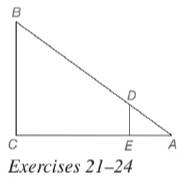Chapter 5.2, Problem 24EElementary Geometry For College St...

7th Edition
Alexander + 2 others
ISBN: 9781337614085

Solutions

Chapter
SectionElementary Geometry For College St...

7th Edition
Alexander + 2 others
ISBN: 9781337614085
Textbook Problem

In Exercises 21 to 24, △ A D E ∼ △ A B C . Given: A D = 4 , A C = 18 , D B = A E Find: A ETo determine

To calculate:

The value of the side AE.

Explanation

Given:

It is given that ΔADEABC.

Definition Used:

Similarity between Two polygons:

Two polygons are similar if and only if when all pairs of corresponding angles are congruent and all pairs of corresponding sides are proportional.

If C is a point of XY¯ and XCY, then XC+CY=XY.

Calculation:

According to the definition of similar polygon, ACAE=BCDE=ABAD.

According to the segment-addition postulate, AC=AE+EC.

Still sussing out bartleby?

Check out a sample textbook solution.

See a sample solution

The Solution to Your Study Problems

Bartleby provides explanations to thousands of textbook problems written by our experts, many with advanced degrees!

Get Started

Sketch the graphs of the equations in Exercises 512. x+y=1

Finite Mathematics and Applied Calculus (MindTap Course List)

Differentiate the function. j(x) = x2.4 + e2.4

Single Variable Calculus: Early Transcendentals, Volume I

In Exercises 49-62, find the indicated limit, if it exists. 55. limx1xx1

Applied Calculus for the Managerial, Life, and Social Sciences: A Brief Approach

The graph at the right has equation:

Study Guide for Stewart's Multivariable Calculus, 8th

y=4x2+1 defines y implicitly as a function of x.

Study Guide for Stewart's Single Variable Calculus: Early Transcendentals, 8th